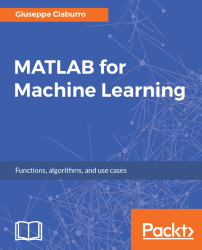•#### MATLAB for Machine Learning#### Overview of this book

MATLAB is the language of choice for many researchers and mathematics experts for machine learning. This book will help you build a foundation in machine learning using MATLAB for beginners. You’ll start by getting your system ready with t he MATLAB environment for machine learning and you’ll see how to easily interact with the Matlab workspace. We’ll then move on to data cleansing, mining and analyzing various data types in machine learning and you’ll see how to display data values on a plot. Next, you’ll get to know about the different types of regression techniques and how to apply them to your data using the MATLAB functions. You’ll understand the basic concepts of neural networks and perform data fitting, pattern recognition, and clustering analysis. Finally, you’ll explore feature selection and extraction techniques for dimensionality reduction for performance improvement. At the end of the book, you will learn to put it all together into real-world cases covering major machine learning algorithms and be comfortable in performing machine learning with MATLAB.
Title PageCreditsForewordwww.PacktPub.comCustomer FeedbackPrefaceFree Chapter
Getting Started with MATLAB Machine LearningImporting and Organizing Data in MATLABFrom Data to Knowledge DiscoveryFinding Relationships between Variables - Regression TechniquesPattern Recognition through Classification AlgorithmsIdentifying Groups of Data Using Clustering MethodsSimulation of Human Thinking - Artificial Neural NetworksImproving the Performance of the Machine Learning Model - Dimensionality ReductionMachine Learning in Practice## Clustering using Gaussian mixture models

So far, we have addressed clustering issues through different approaches. Another way of dealing with these problems is the model-based approach. In this case, we will use certain models for clusters and attempt to optimize the fit between the data and the model. In other words, each cluster can be mathematically represented by a parametric distribution, such as Gaussian (continuous) or Poisson (discrete).

### Gaussian distribution

Gaussian distribution has some limitations when modeling real-world datasets. Very complex densities can be modeled with a linear combination of Gaussian weights weighed appropriately. A mixture model is a type of density model that is packed with a number of density functions, usually Gaussian (Gaussian Mixture Models (GMM)), and these functions are combined to provide multimodal density. These models allow the representation of probability distributions in the presence of subpopulations, where the mixture components are the...